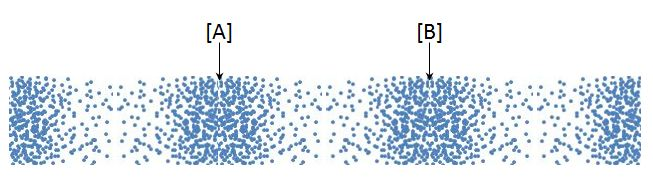# Sound waveLongitudinal waves, also known as "l-waves", are waves in which the displacement of the medium is in the same direction as, or the opposite direction to, the direction of travel of the wave. The above is a schematic diagram of a sound wave at a particular instant, which is a longitudinal wave. [A] and [B] are the most compressed positions and the distance between [A] and [B] is $50$ cm. Which of the following statements is correct?

The velocity of sound is $340$ m/s.

a) The wavelength of this sound is $1$ m.
b) The frequency of this sound is $680$ Hz.
c) After one period passes, position [A] becomes a rarefaction.

×